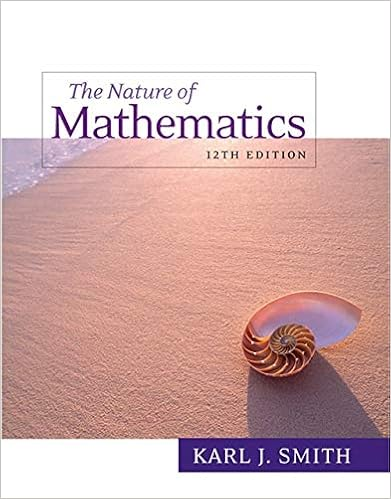# Multiply the integers a 17 8 136 b 623 138 c 41 0 d

• Homework Help
• 3
• 100% (1) 1 out of 1 people found this document helpful

This preview shows page 2 - 3 out of 3 pages.

##### We have textbook solutions for you!
The document you are viewing contains questions related to this textbook.The document you are viewing contains questions related to this textbook.
Chapter 5 / Exercise 8
Nature of Mathematics
SmithExpert Verified
10. Multiply the integers
0
11. Multiply
012. Simplify
b.– 5
13. Divide
b.56
14. One of the most famous blizzards in the United States was the blizzard of 1888. In one part of Nebraska, the temperature plunged from 40°F to −25°F in 5 hr. What was the average change in temperature during this time?
15. A submarine descended from a depth of −528 m to −1804 m in a 2-hr period. What was the average change in depth per hour during this time?
16. Simplify using the order of operations
-4
5 17. Bill's daughter is 30 years younger than he is. Write an expression for his daughter's age if Bill is A years old.
18. Suppose that the amount of rain that fell on Monday was twice the amount that fell on Sunday. Write an expression for the amount of rain on Monday, if Sunday's amount was t inches.
##### We have textbook solutions for you!
The document you are viewing contains questions related to this textbook.The document you are viewing contains questions related to this textbook.
Chapter 5 / Exercise 8
Nature of Mathematics
SmithExpert Verified
Math 040: Re-Entry Mathematics Franklin University19. Write each phrase as an algebraic expressiona.The quotient of t and -7
d.Twice the sum of c and d 2(c+d)20. Evaluate the expression for the given values of the variables.
•••